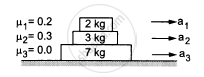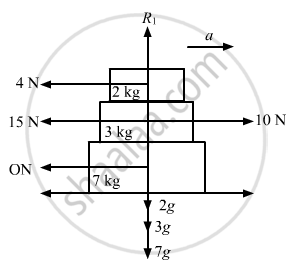Advertisement Remove all ads

# Find the Accelerations A1, A2, A3 of the Three Blocks Shown in the Following Figure If a Horizontal Force of 10 N is Applied on (A) 2 Kg Block, (B) 3 Kg Block, (C) 7 Kg Block. Take G = 10 M/S2. - Physics

Sum

Find the accelerations a1, a2, a3 of the three blocks shown in the following figure if a horizontal force of 10 N is applied on (a) 2 kg block, (b) 3 kg block, (c) 7 kg block. Take g = 10 m/s2.Advertisement Remove all ads

#### Solution

Given:
μ1 = 0.2
μ2 = 0.3
μ3 = 0.4
Using the free body diagram, we have:(a) When the 10 N force is applied to the 2 kg block, it experiences maximum frictional force.
Here,
μ1R1 = μ1 × m1g
μ1R1 = μ1 × 2g = (0.2) × 20
= 4 N              (From the 3 kg block)
Net force experienced by the 2 kg block = 10 − 4 = 6 N

therefore a_1=6/2=3  "m/s"^2

But for the 3 kg block (Fig. 3), the frictional force from the 2 kg block, i.e, 4 N, becomes the driving force and the maximum frictional force between the 3 kg and 7 kg blocks.
Thus, we have:
μ2 = R2 = μ2m2g = (0.3) × 5 kg
= 15 N

Therefore, the 3 kg block cannot move relative to the 7 kg block.
The 3 kg block and the 7 kg block have the same acceleration (a2 = a3), which is due to the 4 N force because there is no friction from the floor.
therefore a_2=a_3=4/10=0.4  "m/s"^2

(b) When the 10 N force is applied to the 3 kg block, it experiences maximum frictional force of (15 + 4) N, i.e., 19 N, from the 2 kg block and the 7 kg block.
So, it cannot move with respect to them.
As the floor is frictionless, all the three bodies move together.
therefore a_1=a_2=a_3=10/12=(5/6)  "m/s"^2

(c) Similarly, it can be proved that when the 10 N force is applied to the 7 kg block, all three blocks move together with the same acceleration.

therefore a_1=a_2=a_3=(5/6)"m/s"^2

Is there an error in this question or solution?
Advertisement Remove all ads

#### APPEARS IN

HC Verma Class 11, 12 Concepts of Physics 1
Chapter 6 Friction
Exercise | Q 23 | Page 98
Advertisement Remove all ads
Advertisement Remove all ads
Share
Notifications

View all notifications

Forgot password?